# bezier.hazmat.triangle_helpers module¶

Pure Python helper methods for bezier.triangle.

bezier.hazmat.triangle_helpers.polynomial_sign(poly_triangle, degree)

Determine the “sign” of a polynomial on the reference triangle.

Note

This is used only by Triangle._compute_valid() (which is in turn used to compute / cache the Triangle.is_valid property).

Checks if a polynomial $$p(s, t)$$ is positive, negative or mixed sign on the reference triangle.

Does this by utilizing the Bézier form of $$p$$: it is a convex combination of the Bernstein basis (real numbers) hence if the Bernstein basis is all positive, the polynomial must be.

If the values are mixed, then we can recursively subdivide until we are in a region where the coefficients are all one sign.

Parameters
• poly_triangle (numpy.ndarray) – 2D array (with 1 row) of control points for a “triangle”, i.e. a bivariate polynomial.

• degree (int) – The degree of the triangle / polynomial given by poly_triangle.

Returns

The sign of the polynomial. Will be one of -1, 1 or 0. A value of 0 indicates a mixed sign or the zero polynomial.

Return type

int

Raises

ValueError – If no conclusion is reached after the maximum number of subdivisions.

bezier.hazmat.triangle_helpers.two_by_two_det(mat)

Compute the determinant of a 2x2 matrix.

This is “needed” because numpy.linalg.det() uses a more generic determinant implementation which can introduce rounding even when the simple $$a d - b c$$ will suffice. For example:

Parameters

mat (numpy.ndarray) – A 2x2 matrix.

Returns

The determinant of mat.

Return type

float

bezier.hazmat.triangle_helpers.quadratic_jacobian_polynomial(nodes)

Compute the Jacobian determinant of a quadratic triangle.

Note

This is used only by Triangle._compute_valid() (which is in turn used to compute / cache the Triangle.is_valid property).

Converts $$\det(J(s, t))$$ to a polynomial on the reference triangle and represents it as a triangle object.

Note

This assumes that nodes is 2 x 6 but doesn’t verify this. (However, the right multiplication by _QUADRATIC_JACOBIAN_HELPER would fail if nodes wasn’t R x 6 and then the ensuing determinants would fail if there weren’t 2 rows.)

Parameters

nodes (numpy.ndarray) – A 2 x 6 array of nodes in a triangle.

Returns

1 x 6 array, coefficients in Bernstein basis.

Return type

numpy.ndarray

bezier.hazmat.triangle_helpers.cubic_jacobian_polynomial(nodes)

Compute the Jacobian determinant of a cubic triangle.

Note

This is used only by Triangle._compute_valid() (which is in turn used to compute / cache the Triangle.is_valid property).

Converts $$\det(J(s, t))$$ to a polynomial on the reference triangle and represents it as a triangle object.

Note

This assumes that nodes is 2 x 10 but doesn’t verify this. (However, the right multiplication by _CUBIC_JACOBIAN_HELPER would fail if nodes wasn’t R x 10 and then the ensuing determinants would fail if there weren’t 2 rows.)

Parameters

nodes (numpy.ndarray) – A 2 x 10 array of nodes in a triangle.

Returns

1 x 15 array, coefficients in Bernstein basis.

Return type

numpy.ndarray

bezier.hazmat.triangle_helpers.de_casteljau_one_round(nodes, degree, lambda1, lambda2, lambda3)

Performs one “round” of the de Casteljau algorithm for triangles.

Note

There is also a Fortran implementation of this function, which will be used if it can be built.

Converts the nodes into a basis for a triangle one degree smaller by using the barycentric weights:

$q_{i, j, k} = \lambda_1 \cdot p_{i + 1, j, k} + \lambda_2 \cdot p_{i, j + 1, k} + \lambda_2 \cdot p_{i, j, k + 1}$
Parameters
• nodes (numpy.ndarray) – The nodes to reduce.

• degree (int) – The degree of the triangle.

• lambda1 (float) – Parameter along the reference triangle.

• lambda2 (float) – Parameter along the reference triangle.

• lambda3 (float) – Parameter along the reference triangle.

Returns

The converted nodes.

Return type

numpy.ndarray

bezier.hazmat.triangle_helpers.make_transform(degree, weights_a, weights_b, weights_c)

Compute matrices corresponding to the de Casteljau algorithm.

Note

This is a helper used only by specialize_triangle().

Applies the de Casteljau to the identity matrix, thus effectively caching the algorithm in a transformation matrix.

Note

This is premature optimization. It’s unclear if the time saved from “caching” one round of de Casteljau is cancelled out by the extra storage required for the 3 matrices.

Parameters
• degree (int) – The degree of a candidate triangle.

• weights_a (numpy.ndarray) – Triple (1D array) of barycentric weights for a point in the reference triangle

• weights_b (numpy.ndarray) – Triple (1D array) of barycentric weights for a point in the reference triangle

• weights_c (numpy.ndarray) – Triple (1D array) of barycentric weights for a point in the reference triangle

Returns

Mapping from keys to the de Casteljau transformation mappings. The keys are 0 corresponding to weights_a, 1 to weights_b and 2 to weights_c.

Return type
bezier.hazmat.triangle_helpers.reduced_to_matrix(shape, degree, vals_by_weight)

Converts a reduced values dictionary into a matrix.

Note

This is a helper used only by specialize_triangle().

The vals_by_weight mapping has keys of the form: (0, ..., 1, ..., 2, ...) where the 0 corresponds to the number of times the first set of barycentric weights was used in the reduction process, and similarly for 1 and 2.

These points correspond to barycentric weights in their own right. For example (0, 0, 0, 1, 2, 2) corresponds to the barycentric weight $$\left(\frac{3}{6}, \frac{1}{6}, \frac{2}{6}\right)$$.

Once the keys in vals_by_weight have been converted to barycentric coordinates, we order them according to our rule (bottom to top, left to right) and then return them in a single matrix.

Parameters
Returns

The newly created reduced control points.

Return type

numpy.ndarray

bezier.hazmat.triangle_helpers.specialize_triangle(nodes, degree, weights_a, weights_b, weights_c)

Specialize a triangle to a reparameterization.

Note

There is also a Fortran implementation of this function, which will be used if it can be built.

Does so by taking three points (in barycentric form) within the reference triangle and then reparameterizing the triangle onto the triangle formed by those three points.

Note

This assumes the triangle is degree 1 or greater but doesn’t check.

Note

This is used only as a helper for subdivide_nodes(), however it may be worth adding this to Triangle as an analogue to Curve.specialize().

Parameters
• nodes (numpy.ndarray) – Control points for a triangle.

• degree (int) – The degree of the triangle.

• weights_a (numpy.ndarray) – Triple (1D array) of barycentric weights for a point in the reference triangle

• weights_b (numpy.ndarray) – Triple (1D array) of barycentric weights for a point in the reference triangle

• weights_c (numpy.ndarray) – Triple (1D array) of barycentric weights for a point in the reference triangle

Returns

The control points for the specialized triangle.

Return type

numpy.ndarray

bezier.hazmat.triangle_helpers.subdivide_nodes(nodes, degree)

Subdivide a triangle into four sub-triangles.

Note

There is also a Fortran implementation of this function, which will be used if it can be built.

Does so by taking the unit triangle (i.e. the domain of the triangle) and splitting it into four sub-triangles by connecting the midpoints of each side.

Parameters
• nodes (numpy.ndarray) – Control points for a triangle.

• degree (int) – The degree of the triangle.

Returns

The nodes for the four sub-triangles.

Return type
bezier.hazmat.triangle_helpers.jacobian_s(nodes, degree, dimension)

Compute $$\frac{\partial B}{\partial s}$$.

Note

This is a helper for jacobian_both(), which has an equivalent Fortran implementation.

Parameters
• nodes (numpy.ndarray) – Array of nodes in a triangle.

• degree (int) – The degree of the triangle.

• dimension (int) – The dimension the triangle lives in.

Returns

Nodes of the Jacobian triangle in Bézier form.

Return type

numpy.ndarray

bezier.hazmat.triangle_helpers.jacobian_t(nodes, degree, dimension)

Compute $$\frac{\partial B}{\partial t}$$.

Note

This is a helper for jacobian_both(), which has an equivalent Fortran implementation.

Parameters
• nodes (numpy.ndarray) – Array of nodes in a triangle.

• degree (int) – The degree of the triangle.

• dimension (int) – The dimension the triangle lives in.

Returns

Nodes of the Jacobian triangle in Bézier form.

Return type

numpy.ndarray

bezier.hazmat.triangle_helpers.jacobian_both(nodes, degree, dimension)

Compute $$s$$ and $$t$$ partial of $$B$$.

Note

There is also a Fortran implementation of this function, which will be used if it can be built.

Parameters
• nodes (numpy.ndarray) – Array of nodes in a triangle.

• degree (int) – The degree of the triangle.

• dimension (int) – The dimension the triangle lives in.

Returns

Nodes of the Jacobian triangles in Bézier form.

Return type

numpy.ndarray

bezier.hazmat.triangle_helpers.jacobian_det(nodes, degree, st_vals)

Compute $$\det(D B)$$ at a set of values.

This requires that $$B \in \mathbf{R}^2$$.

Note

This assumes but does not check that each (s, t) in st_vals is inside the reference triangle.

Warning

This relies on helpers in bezier for computing the Jacobian of the triangle. However, these helpers are not part of the public API and may change or be removed.

>>> nodes = np.asfortranarray([
...     [0.0, 1.0, 2.0, 0.0, 1.5, 0.0],
...     [0.0, 0.0, 0.0, 1.0, 1.5, 2.0],
... ])
>>> triangle = bezier.Triangle(nodes, degree=2)
>>> st_vals = np.asfortranarray([
...     [0.25, 0.0  ],
...     [0.75, 0.125],
...     [0.5 , 0.5  ],
... ])
>>> s_vals, t_vals = st_vals.T
>>> triangle.evaluate_cartesian_multi(st_vals)
array([[0.5 , 1.59375, 1.25 ],
[0.  , 0.34375, 1.25 ]])
>>> # B(s, t) = [s(t + 2), t(s + 2)]
>>> s_vals * (t_vals + 2)
array([0.5 , 1.59375, 1.25 ])
>>> t_vals * (s_vals + 2)
array([0. , 0.34375, 1.25 ])
>>> jacobian_det(nodes, 2, st_vals)
array([4.5 , 5.75, 6. ])
>>> # det(DB) = 2(s + t + 2)
>>> 2 * (s_vals + t_vals + 2)
array([4.5 , 5.75, 6. ])


Note

There is also a Fortran implementation of this function, which will be used if it can be built.

Parameters
• nodes (numpy.ndarray) – Nodes defining a Bézier triangle $$B(s, t)$$.

• degree (int) – The degree of the triangle $$B$$.

• st_vals (numpy.ndarray) – N x 2 array of Cartesian inputs to Bézier triangles defined by $$B_s$$ and $$B_t$$.

Returns

Array of all determinant values, one for each row in st_vals.

Return type

numpy.ndarray

bezier.hazmat.triangle_helpers.classify_tangent_intersection(intersection, nodes1, tangent1, nodes2, tangent2)

Helper for classify_intersection() at tangencies.

Note

This is a helper used only by classify_intersection().

Parameters
• intersection (Intersection) – An intersection object.

• nodes1 (numpy.ndarray) – Control points for the first curve at the intersection.

• tangent1 (numpy.ndarray) – The tangent vector to the first curve at the intersection (2 x 1 array).

• nodes2 (numpy.ndarray) – Control points for the second curve at the intersection.

• tangent2 (numpy.ndarray) – The tangent vector to the second curve at the intersection (2 x 1 array).

Returns

The “inside” curve type, based on the classification enum. Will either be opposed or one of the tangent values.

Return type

IntersectionClassification

Raises

NotImplementedError – If the curves are tangent at the intersection and have the same curvature.

bezier.hazmat.triangle_helpers.ignored_edge_corner(edge_tangent, corner_tangent, corner_previous_edge)

Check ignored when a corner lies inside another edge.

Note

This is a helper used only by ignored_corner(), which in turn is only used by classify_intersection().

Helper for ignored_corner() where one of s and t are 0, but not both.

Parameters
• edge_tangent (numpy.ndarray) – Tangent vector (2 x 1 array) along the edge that the intersection occurs in the middle of.

• corner_tangent (numpy.ndarray) – Tangent vector (2 x 1 array) at the corner where intersection occurs (at the beginning of edge).

• corner_previous_edge (numpy.ndarray) – Edge that ends at the corner intersection (whereas corner_tangent comes from the edge that begins at the corner intersection). This is a 2 x N array where N is the number of nodes on the edge.

Returns

Indicates if the corner intersection should be ignored.

Return type

bool

bezier.hazmat.triangle_helpers.ignored_double_corner(intersection, tangent_s, tangent_t, edge_nodes1, edge_nodes2)

Check if an intersection is an “ignored” double corner.

Note

This is a helper used only by ignored_corner(), which in turn is only used by classify_intersection().

Helper for ignored_corner() where both s and t are 0.

Does so by checking if either edge through the t corner goes through the interior of the other triangle. An interior check is done by checking that a few cross products are positive.

Parameters
Returns

Indicates if the corner is to be ignored.

Return type

bool

bezier.hazmat.triangle_helpers.ignored_corner(intersection, tangent_s, tangent_t, edge_nodes1, edge_nodes2)

Check if an intersection is an “ignored” corner.

Note

This is a helper used only by classify_intersection().

An “ignored” corner is one where the triangles just “kiss” at the point of intersection but their interiors do not meet.

We can determine this by comparing the tangent lines from the point of intersection.

Note

This assumes the intersection has been shifted to the beginning of a curve so only checks if s == 0.0 or t == 0.0 (rather than also checking for 1.0).

Parameters
Returns

Indicates if the corner is to be ignored.

Return type

bool

bezier.hazmat.triangle_helpers.classify_intersection(intersection, edge_nodes1, edge_nodes2)

Determine which curve is on the “inside of the intersection”.

Note

This is a helper used only by Triangle.intersect().

This is intended to be a helper for forming a CurvedPolygon from the edge intersections of two Triangle-s. In order to move from one intersection to another (or to the end of an edge), the interior edge must be determined at the point of intersection.

The “typical” case is on the interior of both edges: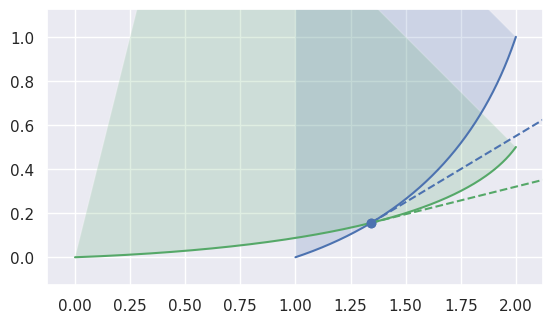>>> nodes1 = np.asfortranarray([
...     [1.0, 1.75, 2.0],
...     [0.0, 0.25, 1.0],
... ])
>>> curve1 = bezier.Curve(nodes1, degree=2)
>>> nodes2 = np.asfortranarray([
...     [0.0, 1.6875, 2.0],
...     [0.0, 0.0625, 0.5],
... ])
>>> curve2 = bezier.Curve(nodes2, degree=2)
>>> s, t = 0.25, 0.5
>>> curve1.evaluate(s) == curve2.evaluate(t)
array([[ True],
[ True]])
>>> tangent1 = hodograph(curve1, s)
>>> tangent1
array([[1.25],
[0.75]])
>>> tangent2 = hodograph(curve2, t)
>>> tangent2
array([[2. ],
[0.5]])
>>> intersection = Intersection(0, s, 0, t)
>>> edge_nodes1 = (nodes1, None, None)
>>> edge_nodes2 = (nodes2, None, None)
>>> classify_intersection(intersection, edge_nodes1, edge_nodes2)
<IntersectionClassification.FIRST: 0>


We determine the interior (i.e. left) one by using the right-hand rule: by embedding the tangent vectors in $$\mathbf{R}^3$$, we compute

$\begin{split}\left[\begin{array}{c} x_1'(s) \\ y_1'(s) \\ 0 \end{array}\right] \times \left[\begin{array}{c} x_2'(t) \\ y_2'(t) \\ 0 \end{array}\right] = \left[\begin{array}{c} 0 \\ 0 \\ x_1'(s) y_2'(t) - x_2'(t) y_1'(s) \end{array}\right].\end{split}$

If the cross product quantity $$B_1'(s) \times B_2'(t) = x_1'(s) y_2'(t) - x_2'(t) y_1'(s)$$ is positive, then the first curve is “outside” / “to the right”, i.e. the second curve is interior. If the cross product is negative, the first curve is interior.

When $$B_1'(s) \times B_2'(t) = 0$$, the tangent vectors are parallel, i.e. the intersection is a point of tangency: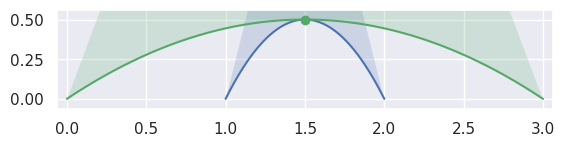>>> nodes1 = np.asfortranarray([
...     [1.0, 1.5, 2.0],
...     [0.0, 1.0, 0.0],
... ])
>>> curve1 = bezier.Curve(nodes1, degree=2)
>>> nodes2 = np.asfortranarray([
...     [0.0, 1.5, 3.0],
...     [0.0, 1.0, 0.0],
... ])
>>> curve2 = bezier.Curve(nodes2, degree=2)
>>> s, t = 0.5, 0.5
>>> curve1.evaluate(s) == curve2.evaluate(t)
array([[ True],
[ True]])
>>> intersection = Intersection(0, s, 0, t)
>>> edge_nodes1 = (nodes1, None, None)
>>> edge_nodes2 = (nodes2, None, None)
>>> classify_intersection(intersection, edge_nodes1, edge_nodes2)
<IntersectionClassification.TANGENT_SECOND: 4>


Depending on the direction of the parameterizations, the interior curve may change, but we can use the (signed) curvature of each curve at that point to determine which is on the interior: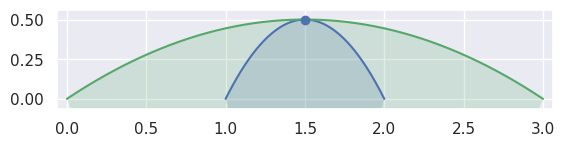>>> nodes1 = np.asfortranarray([
...     [2.0, 1.5, 1.0],
...     [0.0, 1.0, 0.0],
... ])
>>> curve1 = bezier.Curve(nodes1, degree=2)
>>> nodes2 = np.asfortranarray([
...     [3.0, 1.5, 0.0],
...     [0.0, 1.0, 0.0],
... ])
>>> curve2 = bezier.Curve(nodes2, degree=2)
>>> s, t = 0.5, 0.5
>>> curve1.evaluate(s) == curve2.evaluate(t)
array([[ True],
[ True]])
>>> intersection = Intersection(0, s, 0, t)
>>> edge_nodes1 = (nodes1, None, None)
>>> edge_nodes2 = (nodes2, None, None)
>>> classify_intersection(intersection, edge_nodes1, edge_nodes2)
<IntersectionClassification.TANGENT_FIRST: 3>


When the curves are moving in opposite directions at a point of tangency, there is no side to choose. Either the point of tangency is not part of any CurvedPolygon intersection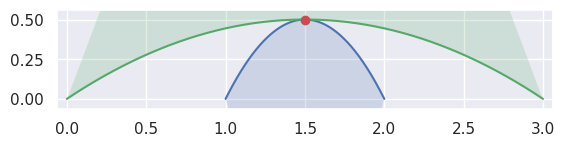>>> nodes1 = np.asfortranarray([
...     [2.0, 1.5, 1.0],
...     [0.0, 1.0, 0.0],
... ])
>>> curve1 = bezier.Curve(nodes1, degree=2)
>>> nodes2 = np.asfortranarray([
...     [0.0, 1.5, 3.0],
...     [0.0, 1.0, 0.0],
... ])
>>> curve2 = bezier.Curve(nodes2, degree=2)
>>> s, t = 0.5, 0.5
>>> curve1.evaluate(s) == curve2.evaluate(t)
array([[ True],
[ True]])
>>> intersection = Intersection(0, s, 0, t)
>>> edge_nodes1 = (nodes1, None, None)
>>> edge_nodes2 = (nodes2, None, None)
>>> classify_intersection(intersection, edge_nodes1, edge_nodes2)
<IntersectionClassification.OPPOSED: 2>


or the point of tangency is a “degenerate” part of two CurvedPolygon intersections. It is “degenerate” because from one direction, the point should be classified as FIRST and from another as SECOND.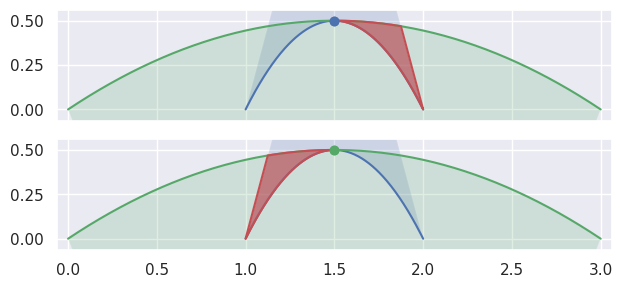>>> nodes1 = np.asfortranarray([
...     [1.0, 1.5, 2.0],
...     [0.0, 1.0, 0.0],
... ])
>>> curve1 = bezier.Curve(nodes1, degree=2)
>>> nodes2 = np.asfortranarray([
...     [3.0, 1.5, 0.0],
...     [0.0, 1.0, 0.0],
... ])
>>> curve2 = bezier.Curve(nodes2, degree=2)
>>> s, t = 0.5, 0.5
>>> curve1.evaluate(s) == curve2.evaluate(t)
array([[ True],
[ True]])
>>> intersection = Intersection(0, s, 0, t)
>>> edge_nodes1 = (nodes1, None, None)
>>> edge_nodes2 = (nodes2, None, None)
>>> classify_intersection(intersection, edge_nodes1, edge_nodes2)
<IntersectionClassification.TANGENT_BOTH: 6>


The TANGENT_BOTH classification can also occur if the curves are “kissing” but share a zero width interior at the point of tangency: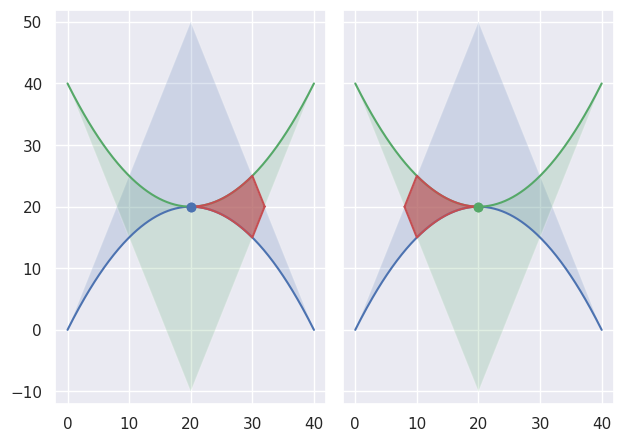>>> nodes1 = np.asfortranarray([
...     [0.0, 20.0, 40.0],
...     [0.0, 40.0,  0.0],
... ])
>>> curve1 = bezier.Curve(nodes1, degree=2)
>>> nodes2 = np.asfortranarray([
...     [40.0, 20.0,  0.0],
...     [40.0,  0.0, 40.0],
... ])
>>> curve2 = bezier.Curve(nodes2, degree=2)
>>> s, t = 0.5, 0.5
>>> curve1.evaluate(s) == curve2.evaluate(t)
array([[ True],
[ True]])
>>> intersection = Intersection(0, s, 0, t)
>>> edge_nodes1 = (nodes1, None, None)
>>> edge_nodes2 = (nodes2, None, None)
>>> classify_intersection(intersection, edge_nodes1, edge_nodes2)
<IntersectionClassification.TANGENT_BOTH: 6>


However, if the curvature of each curve is identical, we don’t try to distinguish further: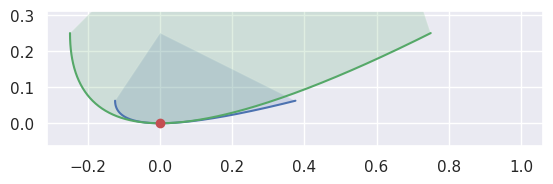>>> nodes1 = np.asfortranarray([
...     [-0.125 , -0.125 , 0.375 ],
...     [ 0.0625, -0.0625, 0.0625],
... ])
>>> curve1 = bezier.Curve(nodes1, degree=2)
>>> nodes2 = np.asfortranarray([
...     [-0.25, -0.25, 0.75],
...     [ 0.25, -0.25, 0.25],
... ])
>>> curve2 = bezier.Curve(nodes2, degree=2)
>>> s, t = 0.5, 0.5
>>> curve1.evaluate(s) == curve2.evaluate(t)
array([[ True],
[ True]])
>>> hodograph(curve1, s)
array([[0.5],
[0. ]])
>>> hodograph(curve2, t)
array([[1.],
[0.]])
>>> curvature(curve1, s)
2.0
>>> curvature(curve2, t)
2.0
>>> intersection = Intersection(0, s, 0, t)
>>> edge_nodes1 = (nodes1, None, None)
>>> edge_nodes2 = (nodes2, None, None)
>>> classify_intersection(intersection, edge_nodes1, edge_nodes2)
Traceback (most recent call last):
...
NotImplementedError: Tangent curves have same curvature.


In addition to points of tangency, intersections that happen at the end of an edge need special handling:>>> nodes1a = np.asfortranarray([
...     [0.0, 4.5, 9.0 ],
...     [0.0, 0.0, 2.25],
... ])
>>> curve1a = bezier.Curve(nodes1a, degree=2)
>>> nodes2 = np.asfortranarray([
...     [11.25, 9.0, 2.75],
...     [ 0.0 , 4.5, 1.0 ],
... ])
>>> curve2 = bezier.Curve(nodes2, degree=2)
>>> s, t = 1.0, 0.375
>>> curve1a.evaluate(s) == curve2.evaluate(t)
array([[ True],
[ True]])
>>> intersection = Intersection(0, s, 0, t)
>>> edge_nodes1 = (nodes1a, None, None)
>>> edge_nodes2 = (nodes2, None, None)
>>> classify_intersection(intersection, edge_nodes1, edge_nodes2)
Traceback (most recent call last):
...
ValueError: ('Intersection occurs at the end of an edge',
's', 1.0, 't', 0.375)
>>>
>>> nodes1b = np.asfortranarray([
...     [9.0, 4.5, 0.0],
...     [2.25, 2.375, 2.5],
... ])
>>> curve1b = bezier.Curve(nodes1b, degree=2)
>>> curve1b.evaluate(0.0) == curve2.evaluate(t)
array([[ True],
[ True]])
>>> intersection = Intersection(1, 0.0, 0, t)
>>> edge_nodes1 = (nodes1a, nodes1b, None)
>>> classify_intersection(intersection, edge_nodes1, edge_nodes2)
<IntersectionClassification.FIRST: 0>


As above, some intersections at the end of an edge are part of an actual intersection. However, some triangles may just “kiss” at a corner intersection: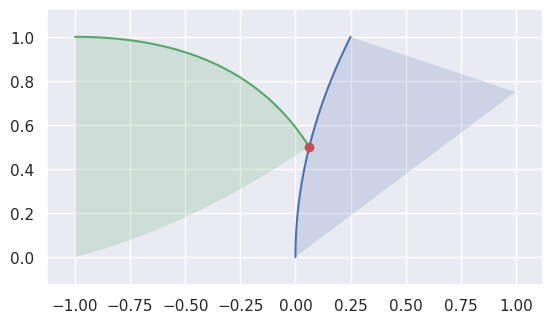>>> nodes1 = np.asfortranarray([
...     [0.25, 0.0, 0.0, 0.625, 0.5  , 1.0 ],
...     [1.0 , 0.5, 0.0, 0.875, 0.375, 0.75],
... ])
>>> triangle1 = bezier.Triangle(nodes1, degree=2)
>>> nodes2 = np.asfortranarray([
...     [0.0625, -0.25, -1.0, -0.5  , -1.0, -1.0],
...     [0.5   ,  1.0 ,  1.0,  0.125,  0.5,  0.0],
... ])
>>> triangle2 = bezier.Triangle(nodes2, degree=2)
>>> curve1, _, _ = triangle1.edges
>>> edge_nodes1 = [curve.nodes for curve in triangle1.edges]
>>> curve2, _, _ = triangle2.edges
>>> edge_nodes2 = [curve.nodes for curve in triangle2.edges]
>>> s, t = 0.5, 0.0
>>> curve1.evaluate(s) == curve2.evaluate(t)
array([[ True],
[ True]])
>>> intersection = Intersection(0, s, 0, t)
>>> classify_intersection(intersection, edge_nodes1, edge_nodes2)
<IntersectionClassification.IGNORED_CORNER: 5>


Note

This assumes the intersection occurs in $$\mathbf{R}^2$$ but doesn’t check this.

Note

This function doesn’t allow wiggle room / round-off when checking endpoints, nor when checking if the cross product is near zero, nor when curvatures are compared. However, the most “correct” version of this function likely should allow for some round off.

Parameters
Returns

The “inside” curve type, based on the classification enum.

Return type

IntersectionClassification

Raises

ValueError – If the intersection occurs at the end of either curve involved. This is because we want to classify which curve to move forward on, and we can’t move past the end of a segment.

bezier.hazmat.triangle_helpers.handle_ends(index1, s, index2, t)

Updates intersection parameters if it is on the end of an edge.

Note

This is a helper used only by Triangle.intersect().

Does nothing if the intersection happens in the middle of two edges.

If the intersection occurs at the end of the first curve, moves it to the beginning of the next edge. Similar for the second curve.

This function is used as a pre-processing step before passing an intersection to classify_intersection(). There, only corners that begin an edge are considered, since that function is trying to determine which edge to move forward on.

Parameters
• index1 (int) – The index (among 0, 1, 2) of the first edge in the intersection.

• s (float) – The parameter along the first curve of the intersection.

• index2 (int) – The index (among 0, 1, 2) of the second edge in the intersection.

• t (float) – The parameter along the second curve of the intersection.

Returns

A triple of:

• flag indicating if the intersection is at the end of an edge

• flag indicating if the intersection is a “corner”

• 4-tuple of the “updated” values (index1, s, index2, t)

Return type
bezier.hazmat.triangle_helpers.to_front(intersection, intersections, unused)

Rotates a node to the “front”.

Note

This is a helper used only by basic_interior_combine(), which in turn is only used by combine_intersections().

If a node is at the end of a segment, moves it to the beginning of the next segment (at the exact same point). We assume that callers have pruned intersections so that there are none with s == 1.0 or t == 1.0. Hence, any such intersection will be an “artificial” intersection added by get_next().

Note

This method checks for exact endpoints, i.e. parameter bitwise identical to 1.0. But it may make sense to allow some wiggle room.

Parameters
Returns

An intersection to (maybe) move to the beginning of the next edge of the triangle.

Return type

Intersection

bezier.hazmat.triangle_helpers.get_next_first(intersection, intersections, to_end=True)

Gets the next node along the current (first) edge.

Note

This is a helper used only by get_next(), which in turn is only used by basic_interior_combine(), which itself is only used by combine_intersections().

Along with get_next_second(), this function does the majority of the heavy lifting in get_next(). Very similar to get_next_second(), but this works with the first curve while the other function works with the second.

Parameters
Returns

point along a triangle of intersection. This will produce the next Optional [ Intersection ] : The “next” intersection along the current (first) edge or the end of the same edge. If to_end is False and there are no other intersections along the current edge, will return None (rather than the end of the same edge).

bezier.hazmat.triangle_helpers.get_next_second(intersection, intersections, to_end=True)

Gets the next node along the current (second) edge.

Note

This is a helper used only by get_next(), which in turn is only used by basic_interior_combine(), which itself is only used by combine_intersections().

Along with get_next_first(), this function does the majority of the heavy lifting in get_next(). Very similar to get_next_first(), but this works with the second curve while the other function works with the first.

Parameters
Returns

point along a triangle of intersection. This will produce the next Optional [ Intersection ] : The “next” intersection along the current (second) edge or the end of the same edge. If to_end is False and there are no other intersections along the current edge, will return None (rather than the end of the same edge).

bezier.hazmat.triangle_helpers.get_next_coincident(intersection, intersections)

Gets the next node along the current (coincident) edge.

Note

This is a helper used only by get_next(), which in turn is only used by basic_interior_combine(), which itself is only used by combine_intersections().

Along with get_next_first() and get_next_second(), this function does the majority of the heavy lifting in get_next().

This function moves immediately to the “next” intersection along the “current” edge. An intersection labeled as COINCIDENT can only occur at the beginning of a segment. The end will correspond to the s or t parameter being equal to 1.0 and so it will get “rotated” to the front of the next edge, where it will be classified according to a different edge pair.

It’s also worth noting that some coincident segments will correspond to curves moving in opposite directions. In that case, there is no “interior” intersection (the curves are facing away) and so that segment won’t be part of an intersection.

Parameters
Returns

The “next” point along a triangle of intersection. This will produce the next intersection along the current (second) edge or the end of the same edge.

Return type

Intersection

bezier.hazmat.triangle_helpers.is_first(classification)

Check if a classification is on the “first” curve.

Parameters

classification (IntersectionClassification) – The classification being checked.

Returns

Indicating if the classification is on the first curve.

Return type

bool

bezier.hazmat.triangle_helpers.is_second(classification)

Check if a classification is on the “second” curve.

Parameters

classification (IntersectionClassification) – The classification being checked.

Returns

Indicating if the classification is on the second curve.

Return type

bool

bezier.hazmat.triangle_helpers.get_next(intersection, intersections, unused)

Gets the next node along a given edge.

Note

This is a helper used only by basic_interior_combine(), which in turn is only used by combine_intersections(). This function does the majority of the heavy lifting for basic_interior_combine().

Note

This function returns Intersection objects even when the point isn’t strictly an intersection. This is “incorrect” in some sense, but for now, we don’t bother implementing a class similar to, but different from, Intersection to satisfy this need.

Parameters
Returns

The “next” point along a triangle of intersection. This will produce the next intersection along the current edge or the end of the current edge.

Return type

Intersection

Raises

ValueError – If the intersection is not classified as FIRST, TANGENT_FIRST, SECOND, TANGENT_SECOND or COINCIDENT.

bezier.hazmat.triangle_helpers.ends_to_curve(start_node, end_node)

Convert a “pair” of intersection nodes to a curve segment.

Note

This is a helper used only by basic_interior_combine(), which in turn is only used by combine_intersections().

Note

This function could specialize to the first or second segment attached to start_node and end_node. We determine first / second based on the classification of start_node, but the callers of this function could provide that information / isolate the base curve and the two parameters for us.

Note

This only checks the classification of the start_node.

Parameters
Returns

The 3-tuple of:

• The edge index along the first triangle (if in {0, 1, 2}) or the edge index along the second triangle shifted to the right by 3 (if in {3, 4, 5})

• The start parameter along the edge

• The end parameter along the edge

Return type
Raises
bezier.hazmat.triangle_helpers.no_intersections(nodes1, degree1, nodes2, degree2)

Determine if one triangle is in the other.

Helper for combine_intersections() that handles the case of no points of intersection. In this case, either the triangles are disjoint or one is fully contained in the other.

To check containment, it’s enough to check if one of the corners is contained in the other triangle.

Parameters
• nodes1 (numpy.ndarray) – The nodes defining the first triangle in the intersection (assumed in :math:mathbf{R}^2).

• degree1 (int) – The degree of the triangle given by nodes1.

• nodes2 (numpy.ndarray) – The nodes defining the second triangle in the intersection (assumed in :math:mathbf{R}^2).

• degree2 (int) – The degree of the triangle given by nodes2.

Returns

Pair (2-tuple) of

Return type
bezier.hazmat.triangle_helpers.tangent_only_intersections(all_types)

Determine intersection in the case of only-tangent intersections.

If the only intersections are tangencies, then either the triangles are tangent but don’t meet (“kissing” edges) or one triangle is internally tangent to the other.

Thus we expect every intersection to be classified as TANGENT_FIRST, TANGENT_SECOND, OPPOSED, IGNORED_CORNER or COINCIDENT_UNUSED.

What’s more, we expect all intersections to be classified the same for a given pairing.

Parameters

all_types (Set [ IntersectionClassification ]) – The set of all intersection classifications encountered among the intersections for the given triangle-triangle pair.

Returns

Pair (2-tuple) of

Return type
Raises
bezier.hazmat.triangle_helpers.basic_interior_combine(intersections, max_edges=10)

Combine intersections that don’t involve tangencies.

Note

This is a helper used only by combine_intersections().

Note

This helper assumes intersections isn’t empty, but doesn’t enforce it.

Parameters
Returns

Pair (2-tuple) of

• List of “edge info” lists. Each list represents a curved polygon and contains 3-tuples of edge index, start and end (see the output of ends_to_curve()).

• ”Contained” boolean. If not None, indicates that one of the triangles is contained in the other.

Return type
Raises

RuntimeError – If the number of edges in a curved polygon exceeds max_edges. This is interpreted as a sign that the algorithm failed.

bezier.hazmat.triangle_helpers.combine_intersections(intersections, nodes1, degree1, nodes2, degree2, all_types)

Combine curve-curve intersections into curved polygon(s).

Note

This is a helper used only by Triangle.intersect().

Does so assuming each intersection lies on an edge of one of two Triangle-s.

Note

This assumes that each intersection has been classified via classify_intersection() and only the intersections classified as FIRST and SECOND were kept.

Parameters
• intersections (List [ Intersection ]) – Intersections from each of the 9 edge-edge pairs from a triangle-triangle pairing.

• nodes1 (numpy.ndarray) – The nodes defining the first triangle in the intersection (assumed in $$\mathbf{R}^2$$).

• degree1 (int) – The degree of the triangle given by nodes1.

• nodes2 (numpy.ndarray) – The nodes defining the second triangle in the intersection (assumed in $$\mathbf{R}^2$$).

• degree2 (int) – The degree of the triangle given by nodes2.

• all_types (Set [ IntersectionClassification ]) – The set of all intersection classifications encountered among the intersections for the given triangle-triangle pair.

Returns

Pair (2-tuple) of

• List of “edge info” lists. Each list represents a curved polygon and contains 3-tuples of edge index, start and end (see the output of ends_to_curve()).

• ”Contained” boolean. If not None, indicates that one of the triangles is contained in the other.

Return type
bezier.hazmat.triangle_helpers.evaluate_barycentric(nodes, degree, lambda1, lambda2, lambda3)

Compute a point on a triangle.

Evaluates $$B\left(\lambda_1, \lambda_2, \lambda_3\right)$$ for a Bézier triangle / triangle defined by nodes.

Note

There is also a Fortran implementation of this function, which will be used if it can be built.

Parameters
• nodes (numpy.ndarray) – Control point nodes that define the triangle.

• degree (int) – The degree of the triangle define by nodes.

• lambda1 (float) – Parameter along the reference triangle.

• lambda2 (float) – Parameter along the reference triangle.

• lambda3 (float) – Parameter along the reference triangle.

Returns

The evaluated point as a D x 1 array (where D is the ambient dimension where nodes reside).

Return type

numpy.ndarray

bezier.hazmat.triangle_helpers.evaluate_barycentric_multi(nodes, degree, param_vals, dimension)

Compute multiple points on the triangle.

Note

There is also a Fortran implementation of this function, which will be used if it can be built.

Parameters
• nodes (numpy.ndarray) – Control point nodes that define the triangle.

• degree (int) – The degree of the triangle define by nodes.

• param_vals (numpy.ndarray) – Array of parameter values (as a N x 3 array).

• dimension (int) – The dimension the triangle lives in.

Returns

The evaluated points, where columns correspond to rows of param_vals and the rows to the dimension of the underlying triangle.

Return type

numpy.ndarray

bezier.hazmat.triangle_helpers.evaluate_cartesian_multi(nodes, degree, param_vals, dimension)

Compute multiple points on the triangle.

Note

There is also a Fortran implementation of this function, which will be used if it can be built.

Parameters
• nodes (numpy.ndarray) – Control point nodes that define the triangle.

• degree (int) – The degree of the triangle define by nodes.

• param_vals (numpy.ndarray) – Array of parameter values (as a N x 2 array).

• dimension (int) – The dimension the triangle lives in.

Returns

The evaluated points, where columns correspond to rows of param_vals and the rows to the dimension of the underlying triangle.

Return type

numpy.ndarray

bezier.hazmat.triangle_helpers.compute_edge_nodes(nodes, degree)

Compute the nodes of each edges of a triangle.

Note

There is also a Fortran implementation of this function, which will be used if it can be built.

Parameters
• nodes (numpy.ndarray) – Control point nodes that define the triangle.

• degree (int) – The degree of the triangle define by nodes.

Returns

The nodes in the edges of the triangle.

Return type
bezier.hazmat.triangle_helpers.shoelace_for_area(nodes)

Compute an auxiliary “shoelace” sum used to compute area.

Note

This is a helper for compute_area().

Defining $$\left[i, j\right] = x_i y_j - y_i x_j$$ as a shoelace term illuminates the name of this helper. On a degree one curve, this function will return

$\frac{1}{2}\left[0, 1\right].$

on a degree two curve it will return

$\frac{1}{6}\left(2 \left[0, 1\right] + 2 \left[1, 2\right] + \left[0, 2\right]\right)$

and so on.

For a given $$\left[i, j\right]$$, the coefficient comes from integrating $$b_{i, d}, b_{j, d}$$ on $$\left[0, 1\right]$$ (where $$b_{i, d}, b_{j, d}$$ are Bernstein basis polynomials).

Returns

The computed sum of shoelace terms.

Return type

float

Raises

UnsupportedDegree – If the degree is not 1, 2, 3 or 4.

bezier.hazmat.triangle_helpers.compute_area(edges)

Compute the area of a curved polygon.

Note

There is also a Fortran implementation of this function, which will be used if it can be built.

Uses Green’s theorem to compute the area exactly. See Triangle.area and CurvedPolygon.area for more information.

Parameters

edges (Tuple [ numpy.ndarray ]) – A list of 2 x N arrays, each of which are control points for an edge of a curved polygon.

Returns

The computed area.

Return type

float